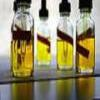#### You may also like### Mathematical Issues for Chemists

A brief outline of the mathematical issues faced by chemistry students.### Reaction Rates

Explore the possibilities for reaction rates versus concentrations with this non-linear differential equation### Catalyse That!

Can you work out how to produce the right amount of chemical in a temperature-dependent reaction?

# Mixing pH

##### Age 16 to 18Challenge Level

”‹”‹”‹”‹”‹”‹The pH of a solution is defined using logarithms as

$$pH = -\log_{10}[H^+],$$
where $[H^+]$ is the concentration of $H^+$ ions in mol/l of the solution.

• Given that the pH of a beaker of pure water is 7, work out how many $H^+$ ions there are in 1 litre of the water.
• A strong acid has a pH of 2. If one litre of this acid is diluted with 1 litre of water, what is the pH of the resulting solution?
• A strong acid has a pH of 1.3. If I have 100ml of this acid, how much water needs to be added to create a solution of pH 2?
• 400ml of an acid of pH 3 is added to 300ml of an acid of pH 4. What is the resulting pH?

Make up some of your own mixing pH questions. For example:

• If I start with $1$ litre of acid of pH $1$, what happens to the pH each time I add $100$ml of water? What sort of curve results?
• Is it the case that when mixing two solutions, the resulting pH is always between the pH of the two initial solutions?